# Kick Tolerance Calculation

This post will teach you how to calculate kick tolerance. This concept is very important to well design and will give you an idea how much kick you can take at particular parameters.

Firstly, I would like to be clear about the following terms;

Kick tolerance is the maximum gas influx volumes (bbl) for a designed kick intensity that can be successfully shut the well in and circulated out without breaking formation pressure at the casing shoe.

Kick intensity is the different between the maximum anticipated formation pressure and planned mud weight.

Secondly, learn how to determin kick tolerance by following steps below:

1. Determine kick intensity

2. Determine maximum allowable shut in casing pressure (MASICP)

3. Determine influx height with this following equation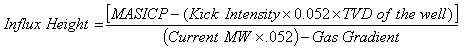4. Calculate influx volume at BHA based on the influx height
Influx volume at BHA, bbl = Kick Height, ft x Annular Capacity of BHA and Hole, bbl/ft

5. Calculate influx volume at shoe base on the influx height
Influx volume at shoe, bbl = Kick Height, ft x Annular Capacity of DP and Hole, bbl/ft

6. Calculate influx volume at the bottom based on the influx volume at the shoe by applying Boyle’s Law

Influx volume at bottom, bbl = (Influx volume at shoe x Leak off test pressure at shoe) ÷ (Formation pressure, psi)

Formation pressure can be calculated from well TVD and maximum anticipated formation pressure.

7. Compare both figures. The smaller figure is the Kick Tolerance.

For more understanding, please determine kick tolerance with following data;

Possible maximum formation pressure = 12.5 ppg
Planned TD mud weight = 12.0 ppg
Casing shoe = 6,500’MD/5,500’ TVD
Leak off test at casing shoe = 14.7 ppg
Hole depth = 10,100’MD/9,500’TVD
Bit = 12-1/4”
BHA = 850 ft
Average OD of BHA = 6.5”
Drill pipe size = 5”
Influx gradient (gas) = 0.11 psi/ft

1. Kick intensity = 12.5 – 12.0 = 0.5 ppg

2. Maximum Allowable Shut In Casing Pressure (MASICP) ** some people may call maximum allowable initial shut in casing pressure**.
= (14.7 – 12.0) x 0.052 x 5,500 = 772 psi

3. Influx height: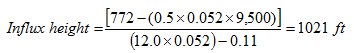4. Calculate influx volume at BHA based on the influx height
Annular capacity between hole and BHA =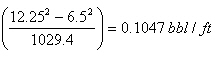Annular capacity between hole and drill pipe  = ( 12.252 – 52) ÷ 1029.4 = 0.1215 bbl/ft
Influx volume at BHA, bbl = (0.1047 x 850) + (0.1215 x 171) = 109.8 bbl
5. Calculate influx volume at shoe base on the influx height
Annular capacity between hole and drill pipe =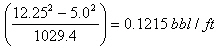Influx volume at shoe, bbl = 0.1215 x 1021 = 124.1 bbl

6. This volume is a reference at shoe; therefore, you need to convert it to the bottom hole condition.

Boyle’s gas law is utilized in order to get the figure at the bottom hole.
P1 x V1 = P2 x V2

For this case, you need to use the maximum pressure at shoe which is the Leak Off Test pressure because it is the maximum value before you will break the wellbore.
Rearranging the equation, you will get like this.

Influx volume at bottom, bbl = Influx volume at shoe x Leak off test pressure at shoe ÷ Formation pressure, psi

Influx volume at shoe = 124.1 bbl
Leak off test pressure = 0.052 x 14.7 x 5,500 = 4,204 psi
Formation pressure = 0.052 x 12.5 x 9,500 = 6,175 psi

Influx volume at bottom, bbl = (124.1 x 4, 204) ÷ (6175) = 84.5 bbl

7. We need to compare 2 cases and the smaller figure is the kick tolerance of the well.
1 st case: 109.8 bbl
2 nd case: 84.5 bbl
Therefore, the kick tolerance is 84.5 bbl.

Conclusion: With the following information, the maximum kick that the well can take and personnel can circulate it out of hole without breaking formation is 84.5 bbl.

Note: Thanks for polwoke checking me some figures.
Ref book: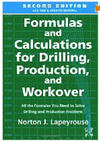Formulas and Calculations for Drilling, Production and Workover, Second Edition

### Related Post

Drill pipe pulled to lose hydrostatic pressure You previously learn about hydrostatic pressure lose due to pulling out of hole . This post will use the same concept but we will determine how many f...
Blow Out Preventer (BOP) Equipment VDO Training BOP equipment is one the most critical equipment for well control operation. Learning about it via VDO training is a good way to understand this subje...
Understand shoe pressure – Shoe pressure when the ... For this case, we will consider the shoe pressure when the gas kick is passing the casing shoe. See the image below. Let’s apply the hydrostatic pr...
Dilution of Mud System to Control Low Gravity Soli... Adding bbl of  drillingfluid can help control low gravity solid (LGS) in mud system. However, this is different from the way to control LGS by adding ...
Share the joy
• 7
•
•
•
•
•
•
•
•
7
SharesWorking in the oil field and loving to share knowledge.

### 15 Responses to Kick Tolerance Calculation

1.polwoker says:

Sir I think there is an error in the influx height calculation when I calculate I get 1021 ft. not 292 ft. I input the exact same values in the equation. please correct me I am wrong. I really appreciate this site really helpful

•DrillingFormulas.Com says:

Thanks for checking it. I correct my figure already.

2.Burnsie says:

Hi there, I couldn’t help but notice that the fore mentioned calculation for influx height does not come to 1021ft but in fact 1041.67ft. Am I missing something here?

•DrillingFormulas.Com says:

Correction gas gradient to 0.11 psi/ft. You will get the 1021 ft.

3.usama says:

Hi there,
I am using this formula from Husain Rabia and getting a different result. i.e 2017.4 ft

H=0.052xρm(TD – CSD) + FGxCSDx0.052–Pf/0.052ρm – G
Correct me if i am wrong.

•DrillingFormulas.Com says:

Hi Usama,

I’ve not expereice with Husain Rabia’s equation. I cannot give you the answer on this one.

Regards,
Syne.

4.Oye says:

Hi there,
Many thanks for this calculation. Your calculation for influx volume at shoe is not very clear, because the length of your BHA is 850ft and the length of your influx is 1021ft, therefore your influx volume at the shoe should be the volume around the drill pipe area and the volume around the BHA area in the open hole. Kindly clarify please? Many thanks.

•DrillingFormulas.Com says:

Oye,

The calculation is update. It is corrected about influx length.

5.RizwanRaz says:

Usama
Result will be same if you are using formula from Hussain Rabia
H= ( 2496+4204-6175 ) / .624-.11
= 1021 ft
As Mud density = 12 ppg Formation density = 12.5 and Frac Density = 14.7
TVD = 9500 ft and CSD = 5500 ft Mud gradient = .624 G = .11

Background concept is same for formula used in above article and for formula used in Hussain Rabia.

•Olu says:

RizwanRaz

I agree with you.

6.Jan says:

I find a error in the Volume calculation at BHA.

The BHA height is 850 ft. Part of the gas will be between DP and Open hole if we wish a column height of 1021.4 ft.
J.

•DrillingFormulas.Com says:

Jan,

The answer is corrected.

7.Bill Burch says:

Only comment on this simplistic approach is that it does not include a safety factor for circulating (usually an operator adds 50-100 psi) nor friction while circulating (it’s assumed that the friction is negligible due to the low rate – ie less than 4 bpm.)

Also, this approach is only valid for water-based mud as it does not account for gas solubility in oil-based muds. It does not include compressibility or thermal effects on the mud system – and therefore the downhole FG does not include the effects either (which are very important in deepwater.)

One small minor error is in the bubble height: since the BHA is only 850 ft long, you must account for the change in geometry. Instead of 106.9 bbls, the correct answer should be 109.9 bbls.

•DrillingFormulas.Com says:

Bill,

Thanks for comment. This is the simple calculation which does not account for any oil based mud effect.

Shyne.

This site uses Akismet to reduce spam. Learn how your comment data is processed.